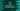# Java program to read user input numbers with blank spaces## Java program to read user input numbers with blank spaces:

In this post, we will learn how to get user-input numbers with blank spaces and add them to an array. We will assume that the input will include only numbers with blank spaces. I will show you different ways to solve this.

### Method 1: By using a loop:

If we know the total count of numbers, we can use a loop to read the numbers to an array. For example:

``````import java.util.Arrays;
import java.util.Scanner;

public class Example {
public static void main(String[] args) {
try (Scanner scanner = new Scanner(System.in)) {
System.out.println("Please enter three numbers with space:");

int[] intArray = new int;
for (int i = 0; i < 3; i++) {
intArray[i] = scanner.nextInt();
}

System.out.println("You have entered: " + Arrays.toString(intArray));
}
}
}``````

Here,

• The program assumes that the user will always enter three numbers, separated with space.
• It created one Scanner object to read the user input values.
• It initialized an integer array `intArray` of size 3 to hold the numbers.
• The `for` loop runs from `i = 0` to `i = 3` and on each iteration, it uses the `nextInt()` method to read the integers. It assigns the numbers to the `intArray`.
• The last line is printing the values of the `intArray`.

If you run this program, it will print outputs as below:

``````Please enter three numbers with space:
22 34 4
You have entered: [22, 34, 4]``````

We can also enter more than three numbers, but it will read only the first three numbers:

``````Please enter three numbers with space:
22 34 4 55 67
You have entered: [22, 34, 4]``````

### Method 2: By split the string:

The above program will work if we know the total count of numbers the user will enter. If we don’t know the count, we can read the input as a `String` and split it to get the numbers. The following example program shows how we can read any amount of user input numbers:

``````import java.util.Arrays;
import java.util.Scanner;

public class Example {
public static void main(String[] args) {
try (Scanner scanner = new Scanner(System.in)) {
System.out.println("Please enter the numbers separated with space:");

String numString = scanner.nextLine();
String[] nums = numString.split(" ");

int[] intArray = new int[nums.length];

for (int i = 0; i < nums.length; i++) {
intArray[i] = Integer.parseInt(nums[i]);
}

System.out.println("You have entered: " + Arrays.toString(intArray));
}
}
}``````

• The `scanner` variable is using the `nextLine()` method to read all the numbers as a string and assigns this value to the `numString` variable.

• The `split()` method will break the string around white spaces and return one array holding the numbers as strings. It is assigned to the `nums` variable.

• The integer array `intArray` is initialized with a length equal to the list `nums`.

• The `for` loop is iterating over the elements of the array `nums`. On each step, it uses the `Integer.parseInt` function to convert the character at `i`th position of the `nums` array to `integer` and assigns it to the `i`th element of the `intArray`.

• The last line is printing the elements of the array `intArray`.

If you run this program, it will print outputs as below:

``````Please enter the numbers separated with space:
1 2 3
You have entered: [1, 2, 3]

Please enter the numbers separated with space:
1 2 3 4 5
You have entered: [1, 2, 3, 4, 5]``````

It will work with any number of elements.